# How to Calculate and Solve for Gas Pore Volume of a Reservoir Fluid Flow | The Calculator Encyclopedia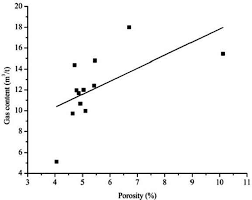The image above represents the gas pore volume.

To compute for the gas pore volume, three essential parameters are needed and these parameters are Initial Gas FVF (Bgi), Initial Water Saturation (Swi) and Initial Gas in Place (G).

The formula for calculating the gas pore volume:

(PV)g = GBgi / 1 – Swi

Where;

(PV)g = Gas Pore Volume
Bgi = Initial Gas FVF
Swi = Initial Water Saturation
G = Initial Gas in Place

Let’s solve an example;
Find the gas pore volume when the initial gas FVF is 12, initial water saturation is 9 and initial gas in place is 13.

This implies that;

Bgi = Initial Gas FVF = 12
Swi = Initial Water Saturation = 9
G = Initial Gas in Place = 13

(PV)g = GBgi / 1 – Swi
(PV)g = 13 x 12 / 1 – 9
(PV)g = 156 / -8
(PV)g =  -19.5

Therefore, the gas pore volume is -19.5

Calculating the Initial Gas FVF when the Gas Pore Volume, Initial Water Saturation and Initial Gas in Place is Given.

Bgi = (PV)g (1 – Swi) / G

Where;

Bgi = Initial Gas FVF
(PV)g = Gas Pore Volume
Swi = Initial Water Saturation
G = Initial Gas in Place

Let’s solve an example;
Find the initial gas FVF when the gas pore volume is 19, initial water saturation is 11 and initial gas in place is 16.

This implies that;

(PV)g = Gas Pore Volume = 19
Swi = Initial Water Saturation = 11
G = Initial Gas in Place = 16

Bgi = (PV)g (1 – Swi) / G
Bgi = 19 (1 – 11) / 16
Bgi = 19 (-10) / 16
Bgi = -190 / 16
Bgi = -11.875

Therefore, the initial gas FVF is -11.875.

Calculating the Initial Water Saturation when the Gas Pore Volume, Initial Gas FVF and Initial Gas in Place is Given.

Swi = 1 – GBgi / (PV)g

Where;

Swi = Initial Water Saturation
G = Initial Gas in Place
(PV)g = Gas Pore Volume
Bgi = Initial Gas FVF

Let’s solve an example;
Find the initial water saturation when the initial gas FVF is 24, initial gas in place is 6 and gas pore volume is 46.

This implies that;

G = Initial Gas in Place = 6
(PV)g = Gas Pore Volume = 46
Bgi = Initial Gas FVF = 24

Swi = 1 – GBgi / (PV)g
Swi = 1 – 6 x 24 / 46
Swi = 1 – 144 / 46
Swi = 1 – 3.13
Swi = -2.13

Therefore, the initial water saturation is -2.13.

Calculating the Initial Gas in Place when the Gas Pore Volume, Initial Gas FVF and  Initial Water Saturation is Given.

G = (PV)g (1 – Swi) / Bgi

Where;

G = Initial Gas in Place
(PV)g = Gas Pore Volume
Bgi = Initial Gas FVF
Swi = Initial Water Saturation

Let’s solve an example;
Find the initial gas in place when the initial gas FVF is 18, initial water saturation is 8 and gas pore volume is 12.

This implies that;

(PV)g = Gas Pore Volume = 12
Bgi = Initial Gas FVF = 18
Swi = Initial Water Saturation = 8

G = (PV)g (1 – Swi) / Bgi
G = 12 (1 – 8) / 18
G = 12 (-7) / 18
G = -84 / 18
G = -4.667

Therefore, the initial gas in place is -4.667.

Nickzom Calculator – The Calculator Encyclopedia is capable of calculating the gas pore volume.

To get the answer and workings of the gas pore volume using the Nickzom Calculator – The Calculator Encyclopedia. First, you need to obtain the app.

You can get this app via any of these means:

To get access to the professional version via web, you need to register and subscribe for NGN 1,500 per annum to have utter access to all functionalities.
You can also try the demo version via https://www.nickzom.org/calculator

Apple (Paid) – https://itunes.apple.com/us/app/nickzom-calculator/id1331162702?mt=8
Once, you have obtained the calculator encyclopedia app, proceed to the Calculator Map, then click on Petroleum under EngineeringNow, Click on Reservoir Fluid Flow under PetroleumNow, Click on gas pore volume under Reservoir Fluid Flow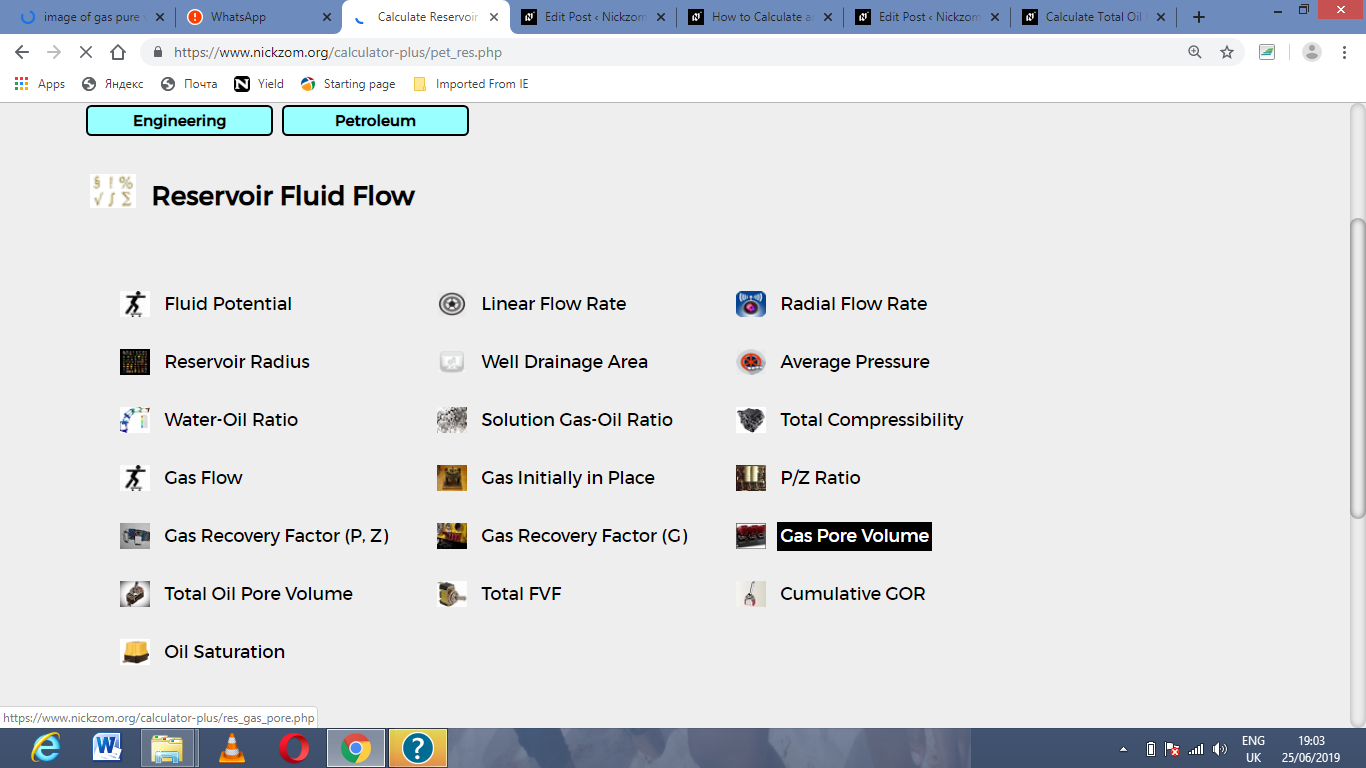The screenshot below displays the page or activity to enter your value, to get the answer for the gas pore volume according to the respective parameter which are the Initial Gas FVF (Bgi), Initial Water Saturation (Swi) and Initial Gas in Place (G).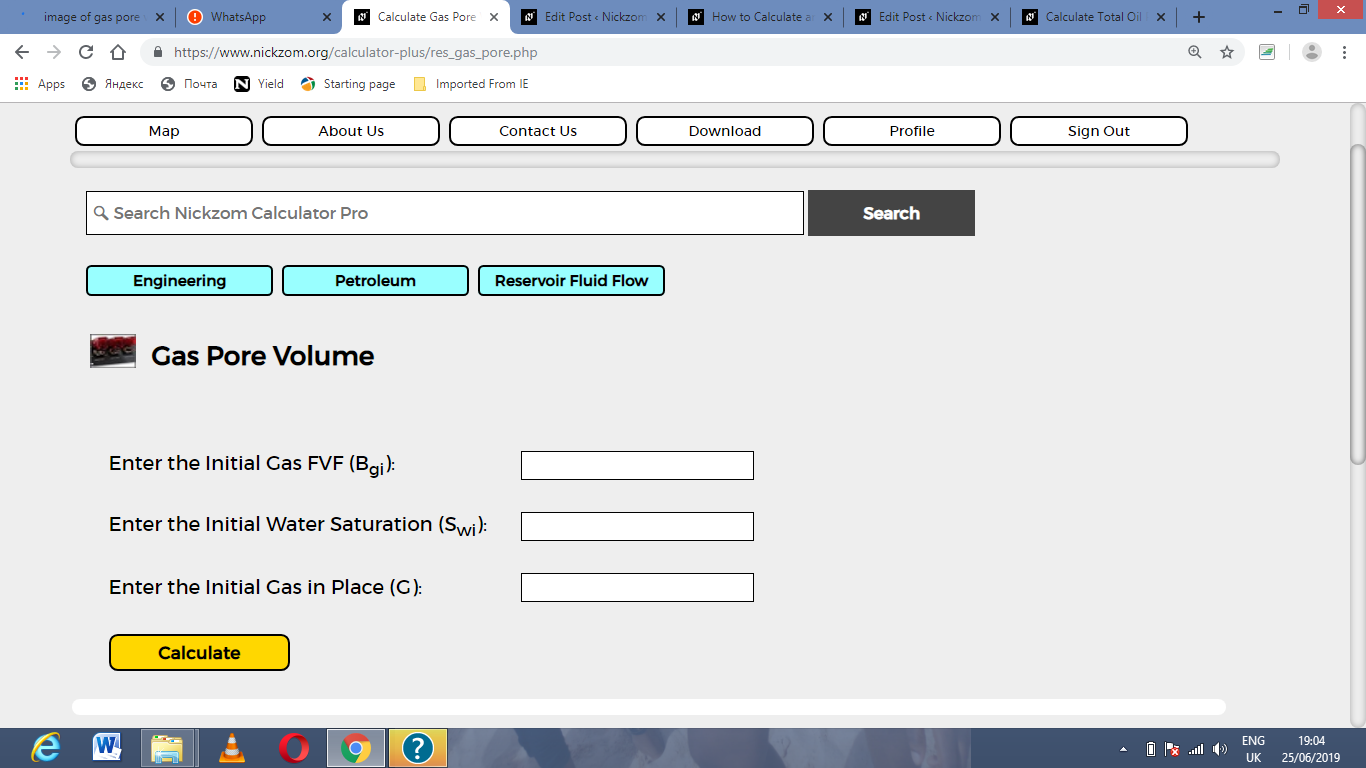Now, enter the value appropriately and accordingly for the parameter as required by the Initial Gas FVF (Bgi) is 12, Initial Water Saturation (Swi) is 9 and Initial Gas in Place (G) is 13.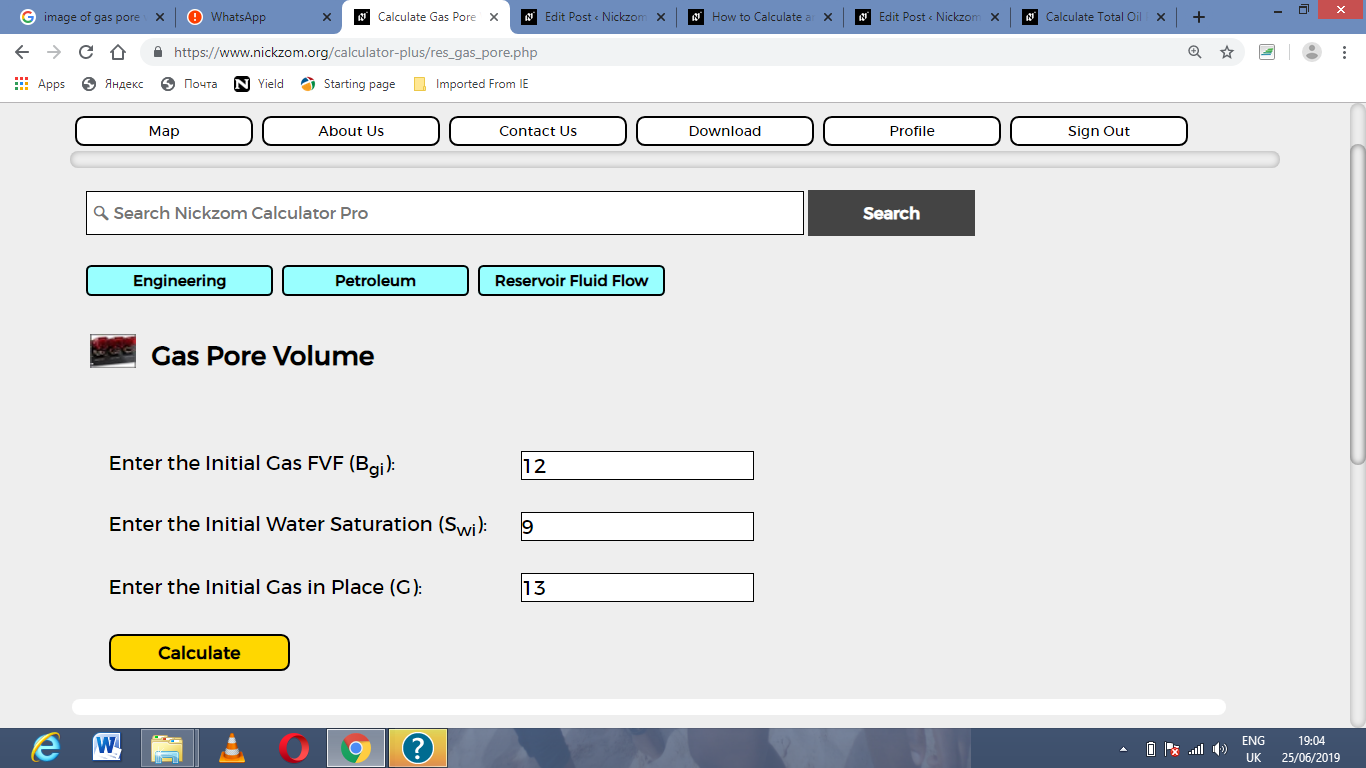Finally, Click on Calculate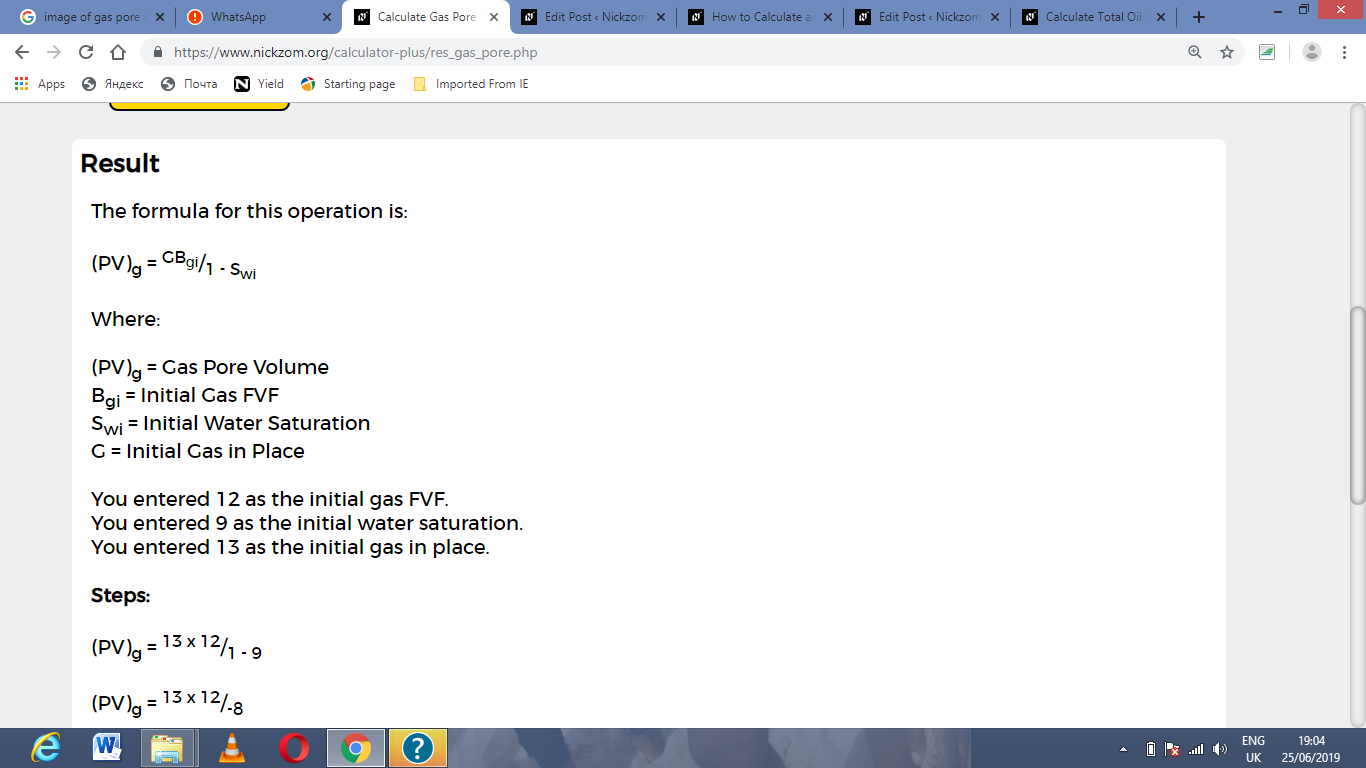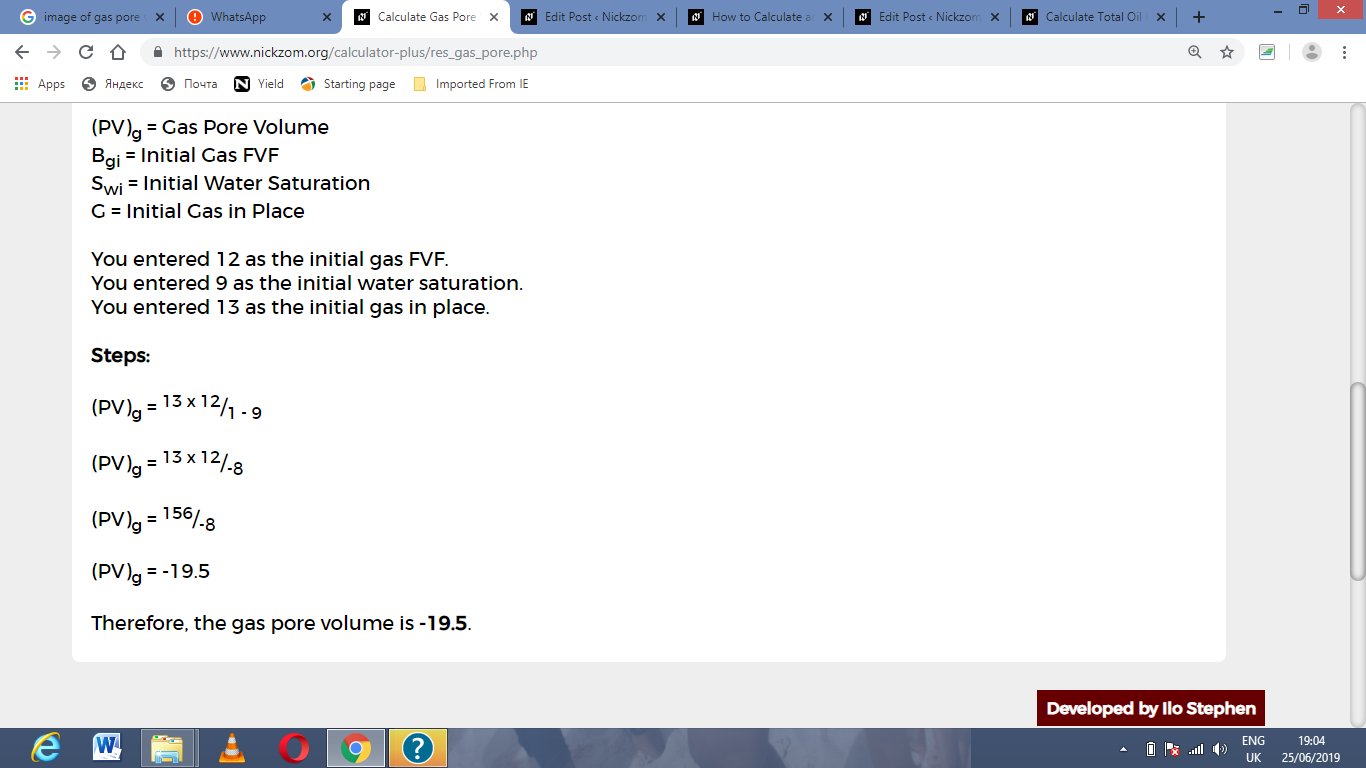As you can see from the screenshot above, Nickzom Calculator– The Calculator Encyclopedia solves for the gas pore volume and presents the formula, workings and steps too.